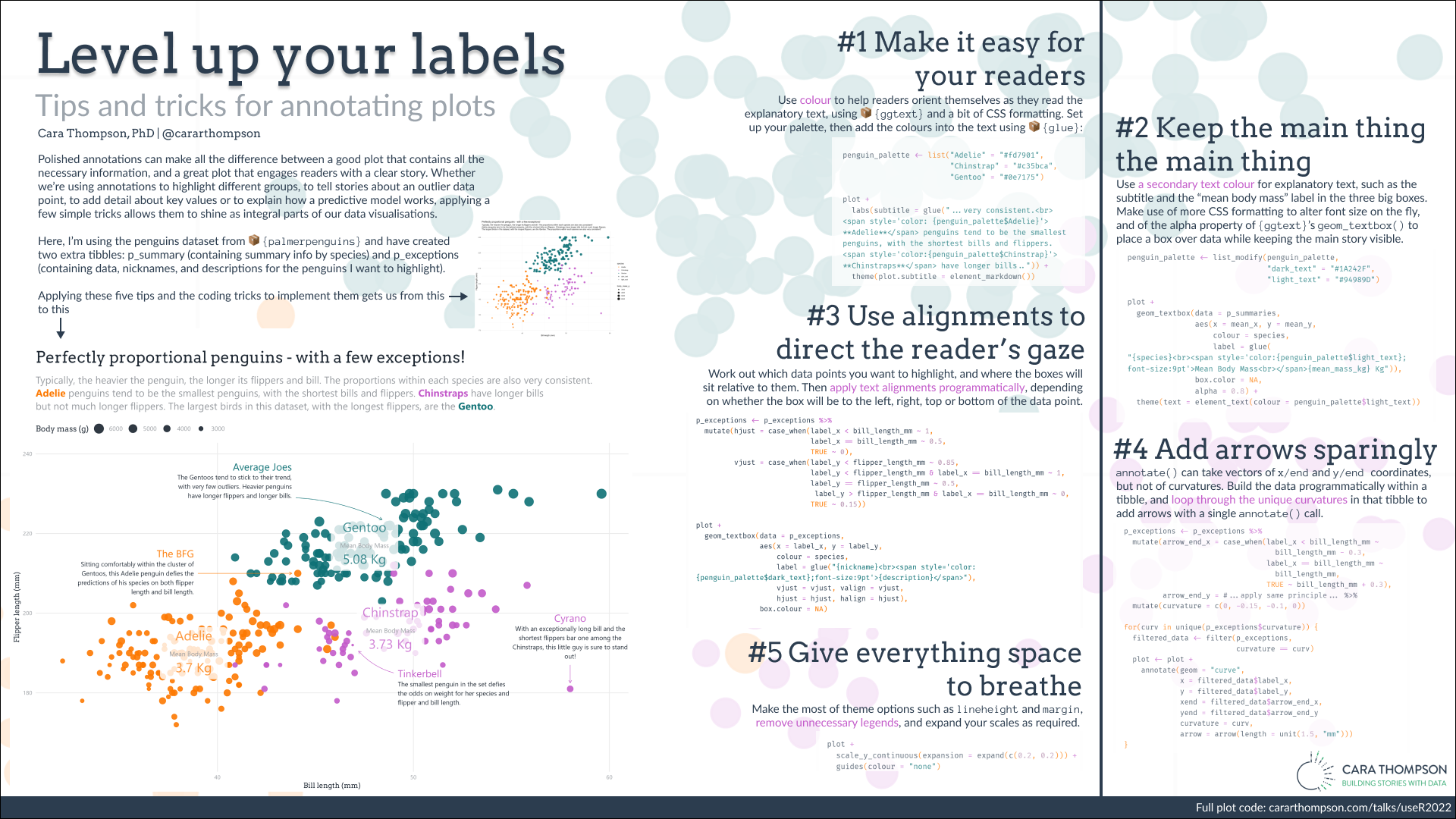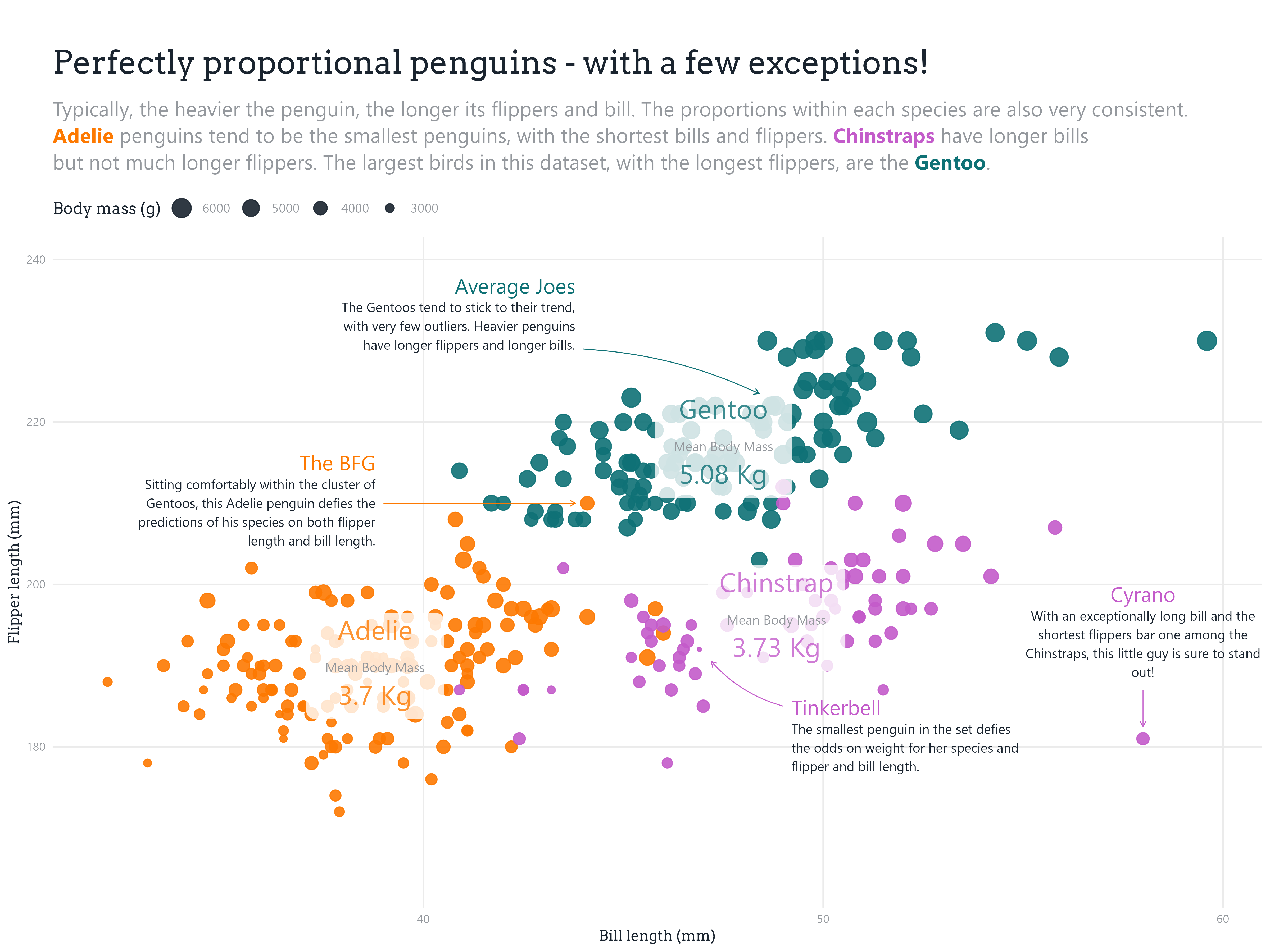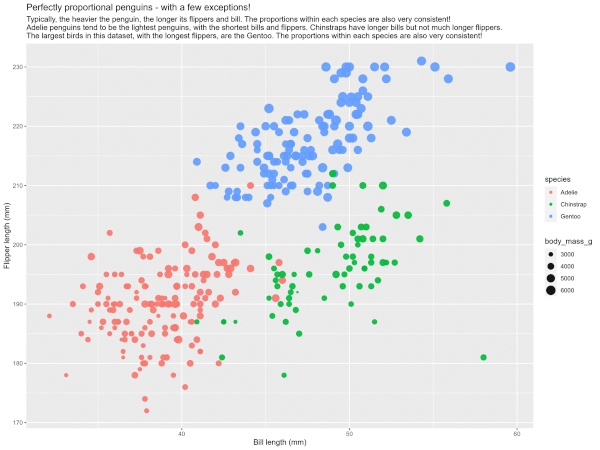# Level Up Your Labels: Tips and Tricks for Annotating Plots

user!2022 - Poster and Elevator Pitch

Cara Thompson
2022-06-22Download the PDF

## Plot code

``````# Libraries ----
library(tidyverse)
library(glue)

# Data source
library(palmerpenguins)

# Annotations
library(extrafont)
library(ggtext)

# Data ----
# |- Penguins ----

penguins <- penguins %>%
# Flipper length and Body mass are integers. We need
# to turn them into numerics to allow the case_when()
# mutations later in the code.
mutate(flipper_length_mm = as.numeric(flipper_length_mm),
body_mass_g = as.numeric(body_mass_g))

penguins %>%
ggplot() +
geom_point(aes(x = bill_length_mm,
y = flipper_length_mm,
colour = species,
size = body_mass_g),
alpha = 0.9) +
labs(title = "Perfectly proportional penguins - with a few exceptions!",
subtitle = "Typically, the heavier the penguin, the longer its flippers and bill. The proportions within each species are also very consistent!
Adelie penguins tend to be the lightest penguins, with the shortest bills and flippers. Chinstraps have longer bills but not much longer flippers.
The largest birds in this dataset, with the longest flippers, are the Gentoo. The proportions within each species are also very consistent!",
x = "Bill length (mm)",
y = "Flipper length (mm)") -> basic_plot

# |- Summary data ----
p_summaries <- penguins %>%
group_by(species) %>%
summarise(mean_mass_kg = round(mean(body_mass_g, na.rm = T)/1000, 2),
mean_x = mean(bill_length_mm, na.rm = T),
mean_y = mean(flipper_length_mm, na.rm = T))

# |- Exceptions ----
p_exceptions <- penguins %>%
filter(bill_length_mm == 48.7 & flipper_length_mm == 222 |
bill_length_mm == 46.9 & flipper_length_mm == 192 |
bill_length_mm == 58.0 & flipper_length_mm == 181 |
bill_length_mm == 44.1 & flipper_length_mm == 210) %>%
arrange(bill_length_mm) %>%
mutate(nickname = c( "The BFG", "Tinkerbell", "Average Joes", "Cyrano"),
description = c("Sitting comfortably within the cluster of Gentoos, this Adelie penguin defies the predictions of his species on both flipper length and bill length.",
"The smallest penguin in the set defies the odds on weight for her species and flipper and bill length.",
"The Gentoos tend to stick to their trend, with very few outliers. Heavier penguins have longer flippers and longer bills.",
"With an exceptionally long bill and the shortest flippers bar one among the Chinstraps, this little guy is sure to stand out!")) %>%
mutate(label_x = c(39, 49, 44, 58),
label_y = c(210, 185, 229,187)) %>%
mutate(label_hjust = case_when(label_x < bill_length_mm ~ 1,
label_x == bill_length_mm ~ 0.5,
TRUE ~ 0),
label_vjust = case_when(label_y < flipper_length_mm ~ 0.85,
label_y < flipper_length_mm &
label_x == bill_length_mm ~ 1,
label_y == flipper_length_mm ~ 0.5,
label_y > flipper_length_mm &
label_x == bill_length_mm ~ 0,
TRUE ~ 0.15)) %>%
mutate(arrow_end_x = case_when(label_x < bill_length_mm ~ bill_length_mm - 0.3,
label_x == bill_length_mm ~ bill_length_mm,
TRUE ~ bill_length_mm + 0.3),
arrow_end_y = case_when(label_y < flipper_length_mm ~ flipper_length_mm - 1.5,
label_y == flipper_length_mm ~ flipper_length_mm,
TRUE ~ flipper_length_mm + 1.5)) %>%
mutate(curvature = c(0, -0.15, -0.1, 0))

# Plot ----
# |- Palette ----
penguin_palette <- list("Adelie" = "#fd7901",
"Chinstrap" = "#c35bca",
"Gentoo" = "#0e7175",
"dark_text" = "#1A242F",
"light_text" = "#94989D")

# To get text colour variants
monochromeR::generate_palette("#1A242F",
"go_lighter",
n_colours = 4)

# |- Main plot ----
labelled_plot <- basic_plot +
scale_colour_manual(values = penguin_palette) +
theme_minimal() +
labs(subtitle = glue("Typically, the heavier the penguin, the longer its flippers and bill.
The proportions within each species are also very consistent.<br>
penguins tend to be the smallest penguins, with the shortest bills
and flippers. <span style='color:{penguin_palette\$Chinstrap}'>**Chinstraps**</span>
have longer bills<br>but not much longer flippers. The largest birds in this dataset,
with the longest flippers, are the
<span style='color:{penguin_palette\$Gentoo}'>**Gentoo**</span>.")) +
geom_textbox(data = p_summaries,
aes(x = mean_x, y = mean_y, colour = species,
label = glue("{species}<span style='color:{penguin_palette\$light_text};
font-size:9pt'><br>Mean Body Mass<br></span>
{mean_mass_kg} Kg")),
halign = 0.5, hjust = 0.5,
size = 6.5,
family = "Segoe UI",
box.color = NA,
alpha = 0.8,
maxwidth = unit(6.5, "lines")) +
geom_textbox(data = p_exceptions,
aes(x = label_x, y = label_y,
colour = species,
vjust = label_vjust, valign = label_vjust,
hjust = label_hjust, halign = label_hjust,
label = glue("{nickname}<br>
<span style='color:{penguin_palette\$dark_text};font-size:9pt'>
{description}</span>")),
width = unit(12, "lines"),
size = 5,
lineheight = 0.9,
fill = NA,
family = "Segoe UI",
box.colour = NA) +
scale_y_continuous(expand = expansion(c(.2, .2))) +
guides(colour = "none",
size = guide_legend(title = "Body mass (g)", reverse = T,
override.aes = list(colour = penguin_palette\$dark_text))) +
theme(text = element_text(family = "Segoe UI", colour = penguin_palette\$light_text),
plot.subtitle = element_markdown(size = 14,
lineheight = 1.3,
margin = unit(c(0, 0, 0.5, 0), "cm")),
plot.title = element_markdown(family = "Arvo",
size = 22, colour = penguin_palette\$dark_text,
margin = unit(c(1, 0, 0.5, 0), "cm")),
axis.text = element_text(colour = penguin_palette\$light_text, size = 8),
axis.title = element_text(family = "Arvo",
colour = penguin_palette\$dark_text, size = 10),
legend.position = "top",
legend.justification = "left",
legend.margin = margin(unit(c(0,-0.45,0,0), "cm")),
legend.title = element_text(family = "Arvo", colour = penguin_palette\$dark_text),
panel.grid.minor = element_blank())

# |- Arrows ----
for(curv in unique(p_exceptions\$curvature)) {

filtered_data <- filter(p_exceptions,
curvature == curv)

labelled_plot <- labelled_plot +
annotate(geom = "curve",
x = filtered_data\$label_x,
y = filtered_data\$label_y,
xend = filtered_data\$arrow_end_x,
yend = filtered_data\$arrow_end_y,
size = 0.3,
colour = case_when(filtered_data\$species == "Adelie" ~ penguin_palette\$Adelie,
filtered_data\$species == "Chinstrap" ~ penguin_palette\$Chinstrap,
filtered_data\$species == "Gentoo" ~ penguin_palette\$Gentoo),
curvature = curv,
arrow = arrow(length = unit(1.5, "mm")))
}

# Export ----
ggsave(labelled_plot, filename = here("annotated_penguins.png"),
dpi = 400, width = 12, height = 9,
bg = "#ffffff")
``````

## Final plotFinal plot produced using the code above

## Making ofGIF showing the transformation of the plot through the steps described in the video

### Citation

For attribution, please cite this work as

`Thompson (2022, June 22). Building stories with data: Level Up Your Labels: Tips and Tricks for Annotating Plots. Retrieved from https://www.cararthompson.com/talks/user2022/`

BibTeX citation

```@misc{thompson2022level,
author = {Thompson, Cara},
title = {Building stories with data: Level Up Your Labels: Tips and Tricks for Annotating Plots},
url = {https://www.cararthompson.com/talks/user2022/},
year = {2022}
}```